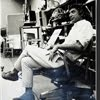## Eugene Ray SDSU 1970'sEugene Ray 1970's. photo credit: Tom Davis

## Monday, February 18, 2013

### JUNGLE wizards= MAYA DESIGN >amazing vision s.of new orleans /one thousnd years of CREATION ^^^^^^^^^^^^^^^^^^^^^ HIGH STRENGTH WIND CHIMES= hydraulic concrete coxcomb roof on fantastic tall pyramid temples ^^^^^^^^^^^^^^^^^^^^^ LONGEST BRIDGE=of ANCIENTS- tall suspension over usamacinta- ^^^^^^^^^^^^^^^^^^^^^ 80 FOOT LONG >OCEAN SHIPS< took maya to louisiana JUNGLES+MAYAN PASSION+
>the former students of my fourth<
>year architecture class at lsu<
>will remember the mayan<
+ARCHITECTURE+
>i stressed in our first project a<
+NATURAL HISTORY MUSEUM+
>in the avery island jungle<
>south of new orleans<
^^^^^^^^^^

+"THE LOST SECRETS OF+
+MAYA TECHNOLOGY"+
>by james a. o'kon, pe<
^^^^^^^^^^^
>engineer out of atlanta georgia<
>is a very revealing reference<
^^^^^^^^^^^^^^^

+AUGUSTUS LE PLONGEON+
>the archaeological seer who found<
>the CHAK MOOL using esp would<
>feel a strong friendship here<
^^^^^^^^^^^^^^^
+THE MAYA SPOKE TO+
+T H E + G O D S+
^^^^^^^
+EUGENE RAY / DEGRUY+
+MAYA YUCATAN+
^^^^^^^^^
>also<
+EUGENE RAY ARCHITECT+
+LE PLONGEON+
^^^^^^^
>when the degruy brothers built the<
+DOMED SUGAR MILL+
>on the banks of the mississippi<
>south of new orleans they<
>were unaware of the<
^^^^^^^^^^^
+MAYAN CONICAL STRUCTURES<
+IN THE YUCATAN JUNGLE+
^^^^^^^^^^^^^^
>w/ wind chime coxcomb roofs<
+HIGH STRENGTH+
+CONCRETE+
>that rose higher then any<
>roman architecture<
^^^^^^^^^^

+TONIGHT'S PHOTOS+
>my degruy ancestors were also<
>long visited new orleans<
^^^^^^^^^^^^
+THE "INDIAN" TEMPLE IN THEIR+
>see photo # five<
+JUNGLE AS VITAL EVIDENCE+
^^^^^^^^^^^^^^^

#1)            +MAYAN CONES+
>rose out of the jungle all over<
>the yucatan, this is at coba<
^^^^^^^^^^^^^^
+SCHOLARS AT THIS POINT+
+"THE GOD'S MACHINES"+
^^^^^^^^^^^^
>to find those cones were more<
>then observatories...they<
>were energy devices<!
^^^^^^^^^^^

#2)          +MAYAN TEMPLES+
>were covered w/ stucco & radiant<
+BRILLIENT COLORS+
>some rising higher then the tower<
>of pisa and the mise-en-scene of<
+FANTASTIC CEREMONIES+
>with<
+FLAMBEAUX TORCHES+
>and the sound of<
^^^^^^^^^
+FLUTES, RATTLES & BELLS+
>powerful synergy<)
^^^^^^^^^^

#3)     +PLATFORM OF CONES+
>("the god's machines" diagram)<
^^^^^^^^^^^^^
>at mayapan near chichen itza is in<
>w. c. bong's energy research a<
^^^^^^^^^^^^^
+GEOMANCY+
>that would have thrilled degruy<
>templar ancestors back in the<
+ORIENT FOREST+
(>magny fouchard chateau<)
^^^^^^^^^^^^^
>eugene ray architect<
>biotronic energy<
^^^^^^^^
+IT ALSO RELATED TO SUGAR+
>award winners at<
>19c new orleans<
+QUALITY in the DEGRUY MILL+
^^^^^^^^^^^^^^^^

#4)       +TENSILE STRUCTURE+
>(lost secrets/maya technology)<
^^^^^^^^^^^^^^^^^
>an amazing maya suspension bridge<
>over the usamacinta river was the<
+LONGEST BRIDGE OF THE+
+ANCIENT WORLD+
^^^^^^^^^
>was as strong as iron<
^^^^^^^^^^^
+AND BROUGHT WEALTH TO+
+19th CENTURY YUCATAN+
^^^^^^^^^^^^^

#5)            +MAYAN SHIPS+
>(lost secrets/mayan technology)<
^^^^^^^^^^^^^^^^^^
>this diagram of a mayan ocean type<
>ship corroborates my previous<
+TRANS MEXICAN GULF+
>conclusion that the degruy<
^^^^^^^^^^^^^^
+INDIAN MOUND TEMPLE+
>was directly influenced from the<
>mayan civilization to the south<
^^^^^^^^^^^^^^^^^
>eugene ray / degruy<
>mayan ships<
^^^^^^

+AUGUSTUS LE PLONGEON IS+
>of masterful research<
^^^^^^^^^^^
+LOST SECRETS OF THE MAYA+
>and<
+THE GODS MACHINES+
>also<
^^
>lets not forget jean lafitte, famed<
>privateer friend of the degruy<
>spent much time in the<
^^^^^^^^^^^^^
+MAYAN YUCATAN+
>i saw a plaque on isla mujeris<
>stating that he died there<
^^^^^^^^^^^^^^
>eugene ray / degruy<
>jean lafitte<
^^^^^^
>let us remember<
^^^^^^^^^
+STARTED IN MY NEW ORLEANS+
>greatly influenced<
+BY THE MAYA YUCATAN+
^^^^^^^^^^^^
>gene ray, lajolla, california<

>eugene ray, mfa, architect<
>professor emeritus, sdsu<
^^^^^^^^^^^^